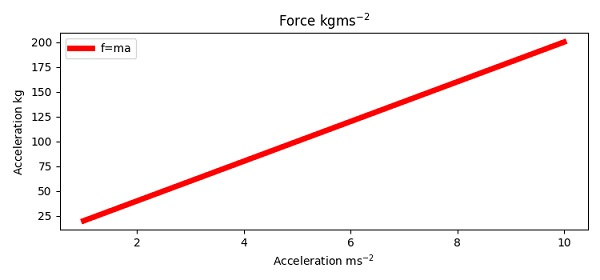# Superscript in Python plots

To put some superscript in Python, we can take the following steps −

• Create points for a and f using numpy.

• Plot f = ma curve using the plot() method, with label f=ma.

• Add title for the plot with superscript, i.e., kgms-2.

• Add xlabel for the plot with superscript, i.e., ms-2.

• Add ylabel for the plot with superscript, i.e., kg.

• To place the legend, use legend() method.

• To display the figure, use the show() method.

## Example

import numpy as np
from matplotlib import pyplot as plt
plt.rcParams["figure.figsize"] = [7.50, 3.50]
plt.rcParams["figure.autolayout"] = True
a = np.linspace(1, 10, 100)
m = 20
f = m*a
plt.plot(a, f, c="red", lw=5, label="f=ma")
plt.title("Force $\mathregular{kgms^{-2}}$")
plt.xlabel("Acceleration $\mathregular{ms^{-2}}$")
plt.ylabel("Acceleration $\mathregular{kg}$")
plt.legend()
plt.show()

## Output